# Exam III Physics 101 Lecture 19 Elasticity and

• Slides: 21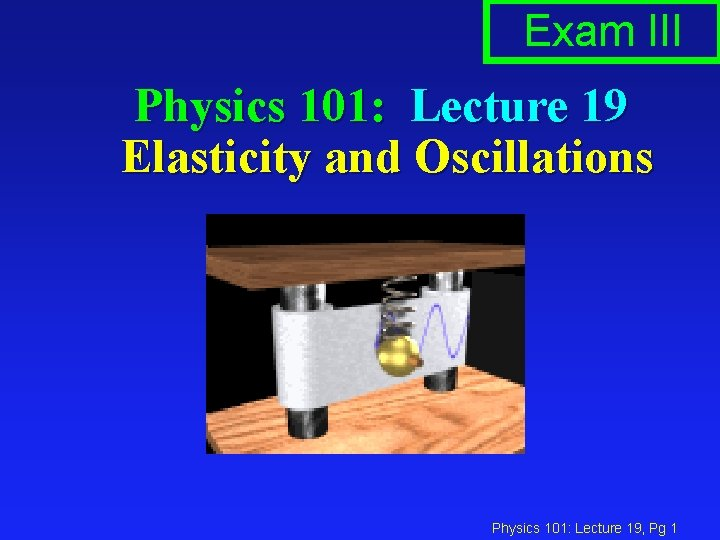Exam III Physics 101: Lecture 19 Elasticity and Oscillations Physics 101: Lecture 19, Pg 1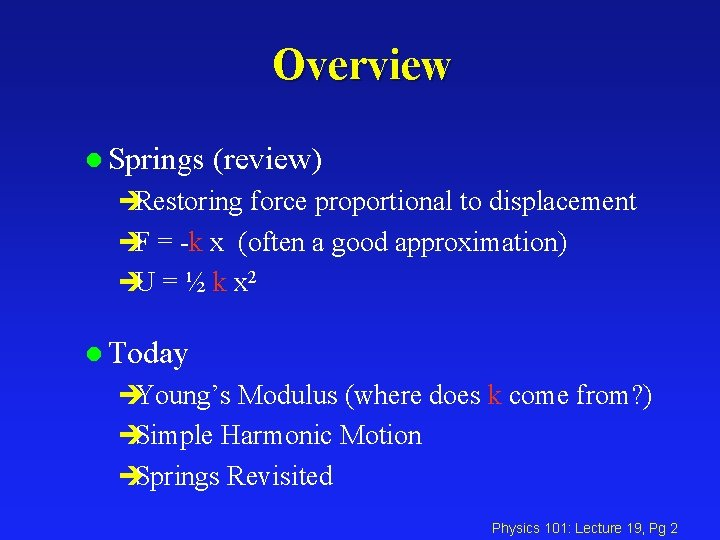Overview l Springs (review) èRestoring force proportional to displacement èF = -k x (often a good approximation) èU = ½ k x 2 l Today èYoung’s Modulus (where does k come from? ) èSimple Harmonic Motion èSprings Revisited Physics 101: Lecture 19, Pg 2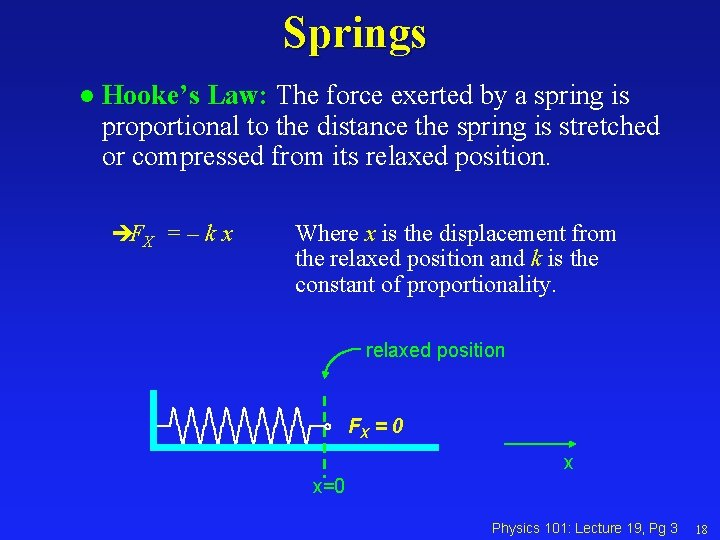Springs l Hooke’s Law: The force exerted by a spring is proportional to the distance the spring is stretched or compressed from its relaxed position. èFX = – k x Where x is the displacement from the relaxed position and k is the constant of proportionality. relaxed position FX = 0 x x=0 Physics 101: Lecture 19, Pg 3 18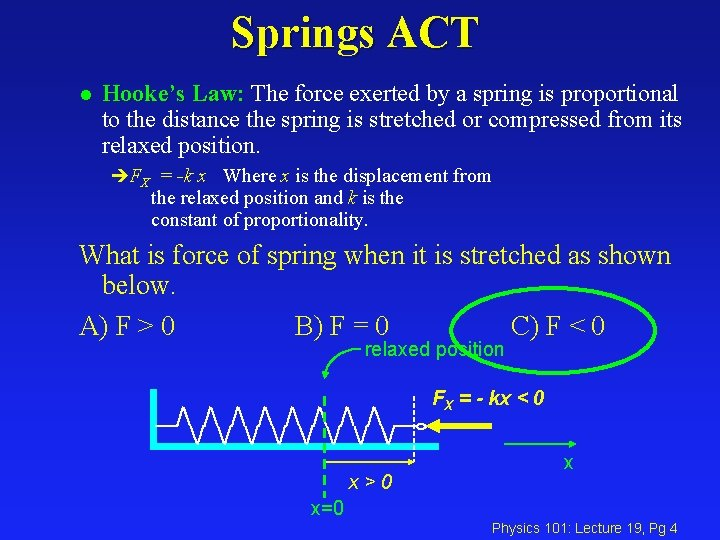Springs ACT l Hooke’s Law: The force exerted by a spring is proportional to the distance the spring is stretched or compressed from its relaxed position. èFX = -k x Where x is the displacement from the relaxed position and k is the constant of proportionality. What is force of spring when it is stretched as shown below. A) F > 0 B) F = 0 C) F < 0 relaxed position FX = - kx < 0 x>0 x x=0 Physics 101: Lecture 19, Pg 4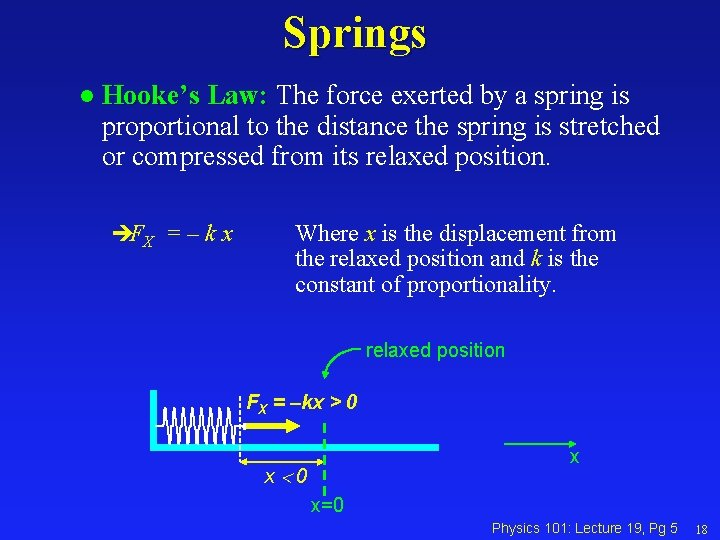Springs l Hooke’s Law: The force exerted by a spring is proportional to the distance the spring is stretched or compressed from its relaxed position. èFX = – k x Where x is the displacement from the relaxed position and k is the constant of proportionality. relaxed position FX = –kx > 0 x x 0 x=0 Physics 101: Lecture 19, Pg 5 18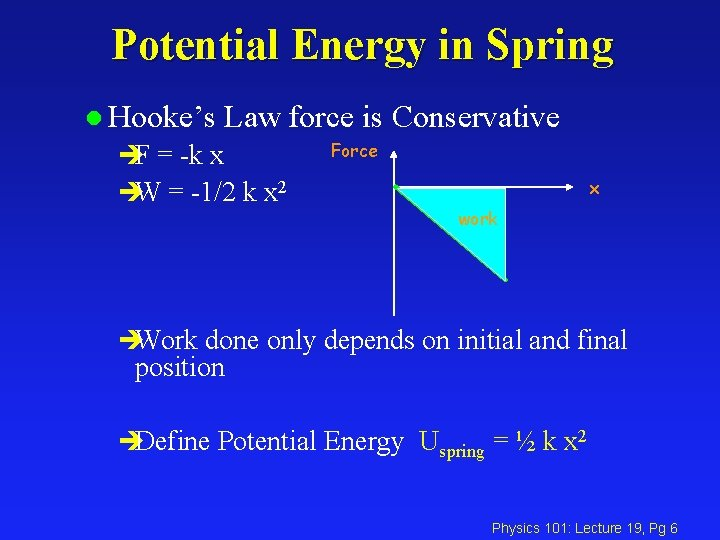Potential Energy in Spring l Hooke’s Law force is Conservative èF = -k x èW = -1/2 k x 2 Force x work èWork done only depends on initial and final position èDefine Potential Energy Uspring = ½ k x 2 Physics 101: Lecture 19, Pg 6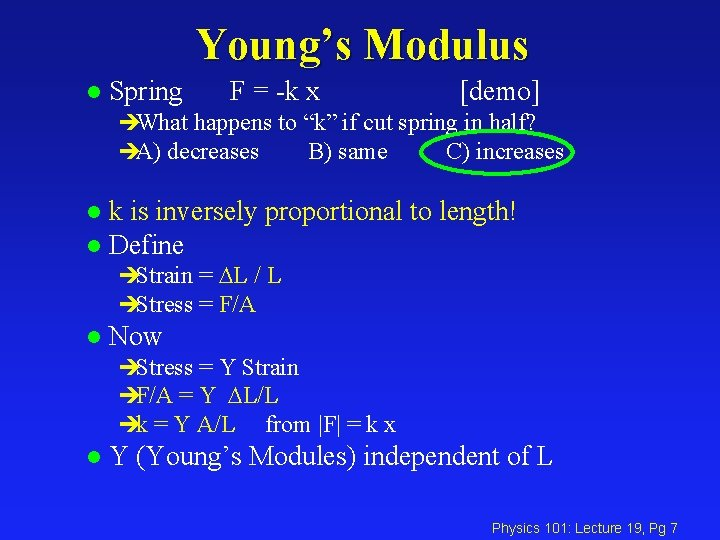Young’s Modulus l Spring F = -k x [demo] èWhat happens to “k” if cut spring in half? èA) decreases B) same C) increases k is inversely proportional to length! l Define l èStrain = DL / L èStress = F/A l Now èStress = Y Strain èF/A = Y DL/L èk = Y A/L from |F| = k x l Y (Young’s Modules) independent of L Physics 101: Lecture 19, Pg 7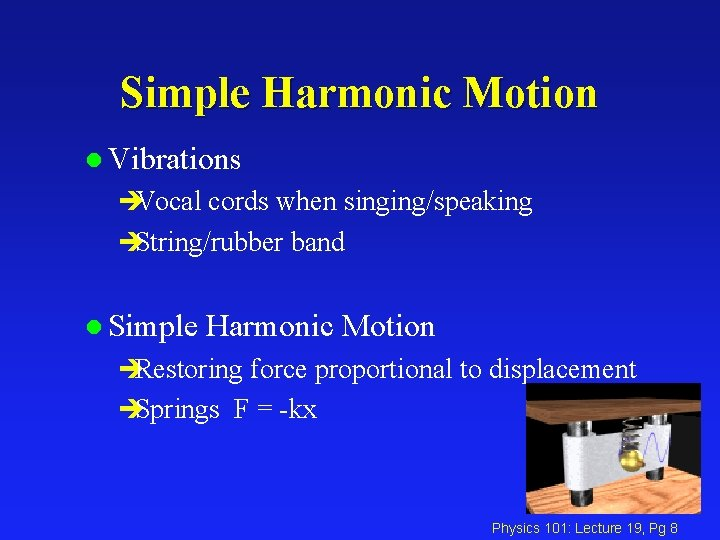Simple Harmonic Motion l Vibrations èVocal cords when singing/speaking èString/rubber band l Simple Harmonic Motion èRestoring force proportional to displacement èSprings F = -kx Physics 101: Lecture 19, Pg 8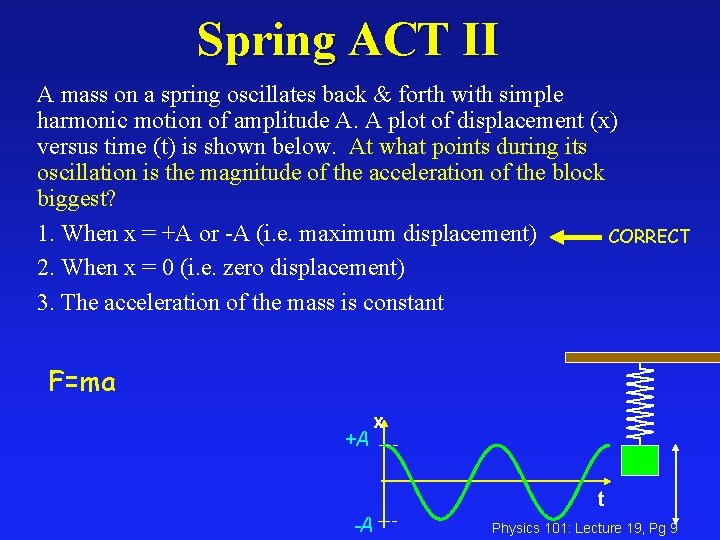Spring ACT II A mass on a spring oscillates back & forth with simple harmonic motion of amplitude A. A plot of displacement (x) versus time (t) is shown below. At what points during its oscillation is the magnitude of the acceleration of the block biggest? 1. When x = +A or -A (i. e. maximum displacement) CORRECT 2. When x = 0 (i. e. zero displacement) 3. The acceleration of the mass is constant F=ma +A x t -A Physics 101: Lecture 19, Pg 9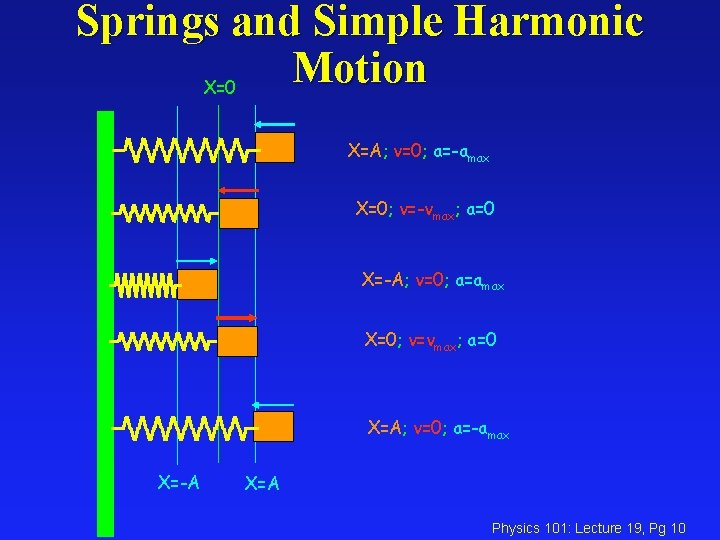Springs and Simple Harmonic Motion X=0 X=A; v=0; a=-amax X=0; v=-vmax; a=0 X=-A; v=0; a=amax X=0; v=vmax; a=0 X=A; v=0; a=-amax X=-A X=A Physics 101: Lecture 19, Pg 10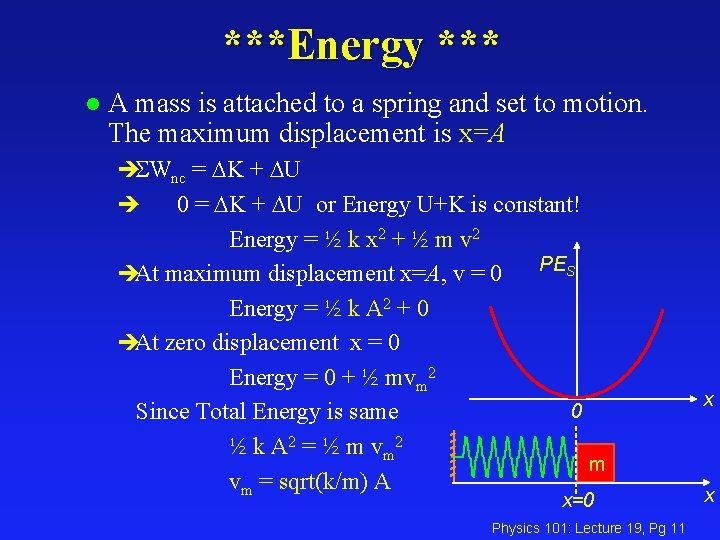***Energy *** l A mass is attached to a spring and set to motion. The maximum displacement is x=A èSWnc = DK + DU 0 = DK + DU or Energy U+K is constant! Energy = ½ k x 2 + ½ m v 2 PES èAt maximum displacement x=A, v = 0 Energy = ½ k A 2 + 0 èAt zero displacement x = 0 Energy = 0 + ½ mvm 2 0 Since Total Energy is same ½ k A 2 = ½ m v m 2 m vm = sqrt(k/m) A è x=0 Physics 101: Lecture 19, Pg 11 x x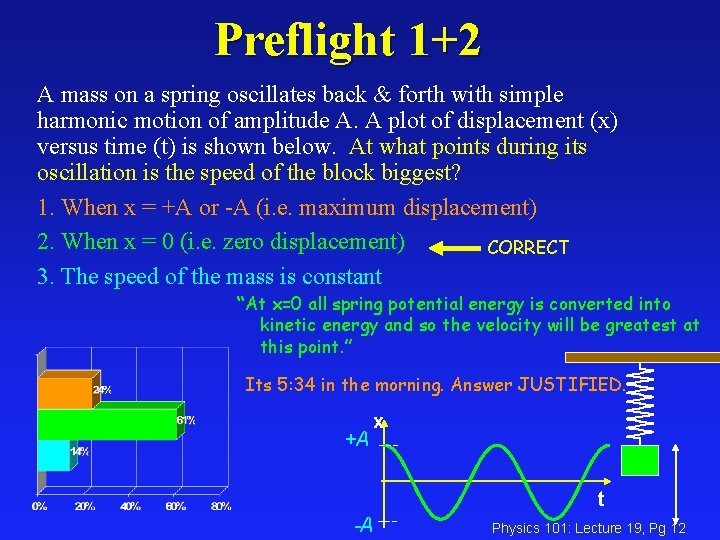Preflight 1+2 A mass on a spring oscillates back & forth with simple harmonic motion of amplitude A. A plot of displacement (x) versus time (t) is shown below. At what points during its oscillation is the speed of the block biggest? 1. When x = +A or -A (i. e. maximum displacement) 2. When x = 0 (i. e. zero displacement) CORRECT 3. The speed of the mass is constant “At x=0 all spring potential energy is converted into kinetic energy and so the velocity will be greatest at this point. ” Its 5: 34 in the morning. Answer JUSTIFIED. +A x t -A Physics 101: Lecture 19, Pg 12Preflight 3+4 A mass on a spring oscillates back & forth with simple harmonic motion of amplitude A. A plot of displacement (x) versus time (t) is shown below. At what points during its oscillation is the total energy (K+U) of the mass and spring a maximum? (Ignore gravity). 1. When x = +A or -A (i. e. maximum displacement) 2. When x = 0 (i. e. zero displacement) 3. The energy of the system is constant. CORRECT The energy changes from spring to kinetic but is not lost. +A x t -A Physics 101: Lecture 19, Pg 13What does moving in a circle have to do with moving back & forth in a straight line ? ? x = R cos q = R cos ( t) since q = w t x x 1 2 y R 3 R 8 q 7 4 6 5 0 -R 1 2 8 7 3 4 6 5 Physics 101: Lecture 19, Pg 14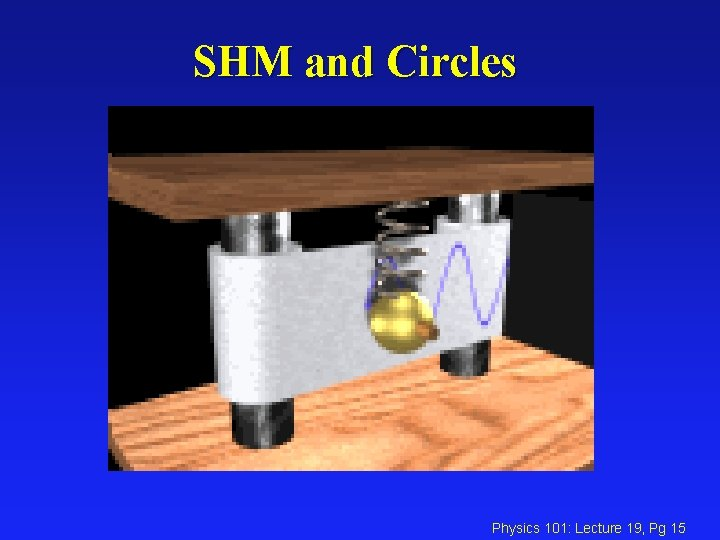SHM and Circles Physics 101: Lecture 19, Pg 15Simple Harmonic Motion: x(t) = [A]cos( t) v(t) = -[A ]sin( t) a(t) = -[A 2]cos( t) x(t) = [A]sin( t) OR v(t) = [A ]cos( t) a(t) = -[A 2]sin( t) xmax = A Period = T (seconds per cycle) vmax = A Frequency = f = 1/T (cycles per second) amax = A 2 Angular frequency = = 2 f = 2 /T For spring: 2 = k/m Physics 101: Lecture 19, Pg 16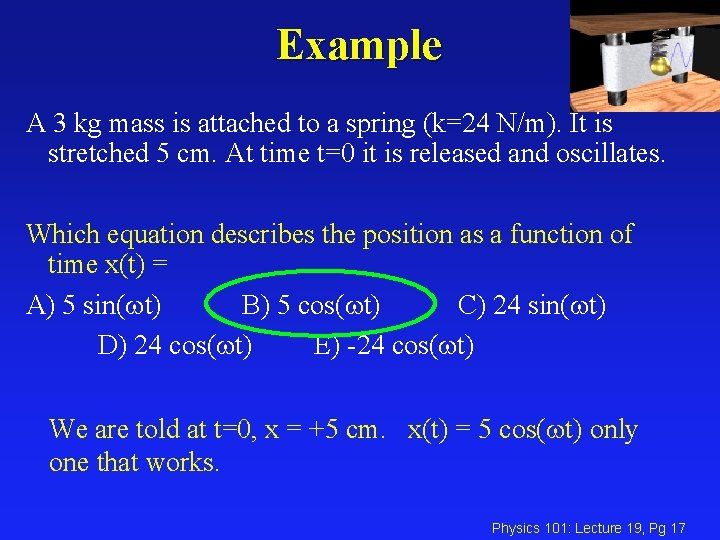Example A 3 kg mass is attached to a spring (k=24 N/m). It is stretched 5 cm. At time t=0 it is released and oscillates. Which equation describes the position as a function of time x(t) = A) 5 sin( t) B) 5 cos( t) C) 24 sin( t) D) 24 cos( t) E) -24 cos( t) We are told at t=0, x = +5 cm. x(t) = 5 cos( t) only one that works. Physics 101: Lecture 19, Pg 17Example A 3 kg mass is attached to a spring (k=24 N/m). It is stretched 5 cm. At time t=0 it is released and oscillates. What is the total energy of the block spring system? A) 0. 03 J B). 05 J C). 08 J E=U+K At t=0, x = 5 cm and v=0: E = ½ k x 2 + 0 = ½ (24 N/m) (5 cm)2 = 0. 03 J Physics 101: Lecture 19, Pg 18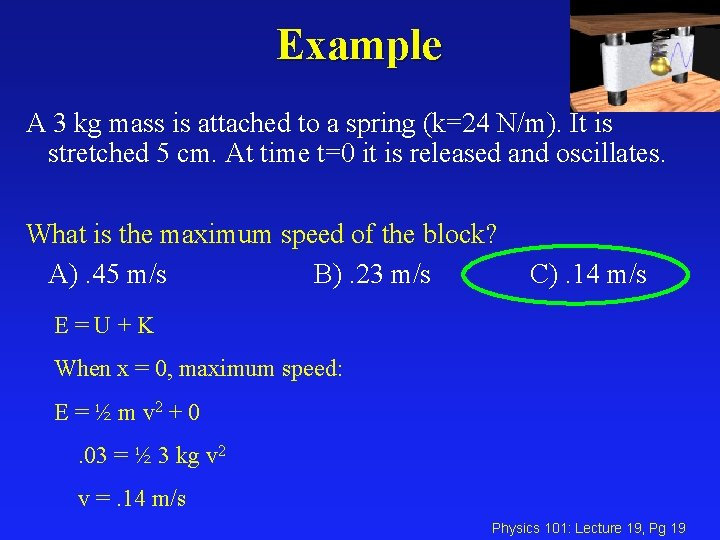Example A 3 kg mass is attached to a spring (k=24 N/m). It is stretched 5 cm. At time t=0 it is released and oscillates. What is the maximum speed of the block? A). 45 m/s B). 23 m/s C). 14 m/s E=U+K When x = 0, maximum speed: E = ½ m v 2 + 0. 03 = ½ 3 kg v 2 v =. 14 m/s Physics 101: Lecture 19, Pg 19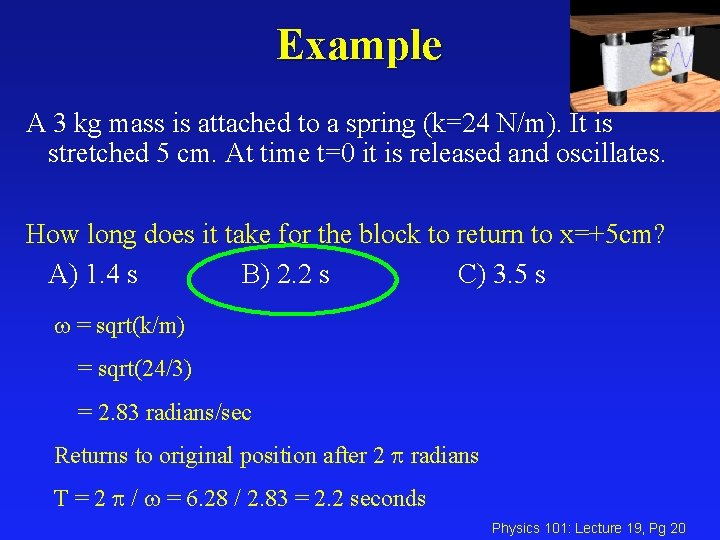Example A 3 kg mass is attached to a spring (k=24 N/m). It is stretched 5 cm. At time t=0 it is released and oscillates. How long does it take for the block to return to x=+5 cm? A) 1. 4 s B) 2. 2 s C) 3. 5 s = sqrt(k/m) = sqrt(24/3) = 2. 83 radians/sec Returns to original position after 2 radians T = 2 / = 6. 28 / 2. 83 = 2. 2 seconds Physics 101: Lecture 19, Pg 20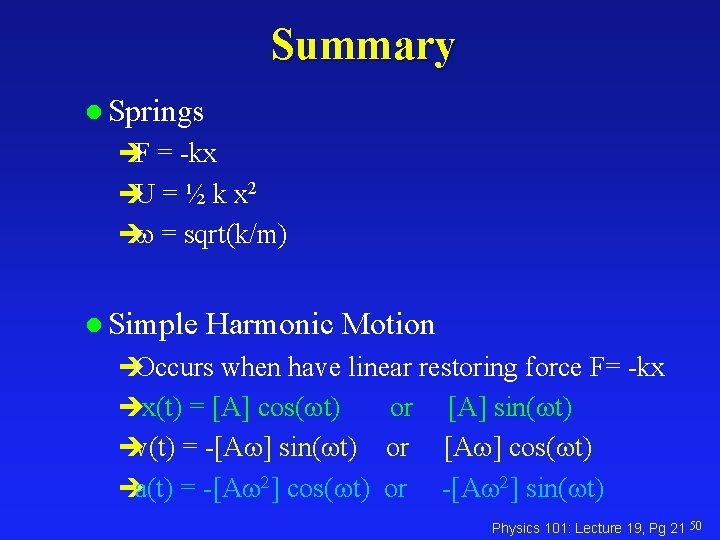Summary l Springs èF = -kx èU = ½ k x 2 è = sqrt(k/m) l Simple Harmonic Motion èOccurs when have linear restoring force F= -kx èx(t) = [A] cos( t) or èv(t) = -[A ] sin( t) or èa(t) = -[A 2] cos( t) or [A] sin( t) [A ] cos( t) -[A 2] sin( t) Physics 101: Lecture 19, Pg 21 50